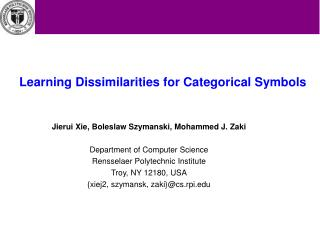Download PresentationJierui Xie, Boleslaw Szymanski, Mohammed J. Zaki Department of Computer Science

# Jierui Xie, Boleslaw Szymanski, Mohammed J. Zaki Department of Computer Science - PowerPoint PPT PresentationDownload Presentation## Jierui Xie, Boleslaw Szymanski, Mohammed J. Zaki Department of Computer Science

- - - - - - - - - - - - - - - - - - - - - - - - - - - E N D - - - - - - - - - - - - - - - - - - - - - - - - - - -
##### Presentation Transcript

1. Learning Dissimilarities for Categorical Symbols Jierui Xie, Boleslaw Szymanski, Mohammed J. Zaki Department of Computer Science Rensselaer Polytechnic Institute Troy, NY 12180, USA {xiej2, szymansk, zaki}@cs.rpi.edu

2. Presentation Outline • Introduction • Related Work • Learning Dissimilarity (LD) Algorithm • Experimental Results • Conclusion

3. Introduction • Distance plays an important role in many data mining tasks • Distance is rarely defined precisely for categorical data • nominal and ordinal • e.g., rating of a movie {very bad, bad, fair, good, very good} • Goal: derive dissimilarities between categorical symbols • To enable the full power of distance-based methods. • Hopefully easier for interpretation as well.

4. Notation • A dataset X ={x1,x2,…,xt} of t data points. Each point xi has m attributes values xi = (x1i,…, xmi ). • Each attribute Ai is drawn from ni discrete values {ai1,…, aini}. Each aij is also called a symbol. • The similarity between symbols aik and ail : • The dissimilarity or • The distance between two data points xi and xj is defined in terms of the distance between symbols

5. Notation (cont.) • Let the frequency of symbol ai in the dataset be then the probability • Class label • Output of the classier on point xi : • The error of misclassifying point xi: • Total classification error:

6. Related Work • Unsupervised methods: • Assign based on frequency; Emphasize mismatch or match for frequent or rare symbols from certain probability or information theory point of views. • Lin • Burnaby • Smirnov • Goodall • Supervised methods: • Take the classes information into account • Value Difference Metric (VDM) • Cheng et al.. • Gambaryan • Eskin • Occurrence Frequency (OF) • Inverse Occurrence Frequency (IOF)

7. Unsupervised Method Examples • Goodall : less frequent attribute values make greater contribution to the overall similarity than frequent attribute values on match. That is, if ai=aj otherwise, 0 • Inverse Occurrence Frequency (IOF): assigns higher weight to mismatches on less frequent symbols. That is, if ai!=aj otherwise, 1

8. Supervised Method Examples • VDM: • Symbols are similar if they occur with a similar relative frequency for all the classes. where Cai,c is the number of times symbol ai occurs in class c. Cai is the total number of times ai occurs in the whole dataset. h is a constant. • Cheng: • based on RBF classier • They attempt to evaluate all the pair-wise distances between symbols, and they optimize the error function using gradient descent method

9. Learning Dissimilarity Algorithm • Motivation: • learn a mapping function from each categorical attribute Ai onto the real number interval based on the classes information may facilitate the classification task and is possible.

10. Learning Dissimilarity Algorithm (cont.) • Based on nearest neighbor classifier and the distancedifference from two classes • Iteration learning • Guided by gradient descent method to minimize the total classification error

11. Learning Dissimilarity Algorithm (cont.) • Objective Function and Update Equation

12. Learning Dissimilarity Algorithm (cont.) • The Derivative of ∆d • The full update equation

13. Learning Dissimilarity Algorithm (cont.) • Intuitive meaning of assignment update

14. Experimental Result • Datasets

15. Experimental Result (cont.) • Redundancy among symbols

16. Experimental Result (cont.) • Comparison with Various Data-Driven Methods • On average, the LD and VDM achieve the best accuracy, indicating that supervised dissimilarities attain better results over the unsupervised ones. Among the unsupervised measures, IOF, Lin are slightly superior to others.

17. Experimental Result (cont.) • Analysis with confidence interval (accuracy +/- standard deviation) • LD performed statistically worse than Lin on datasets Splice and Tic-tac-toe but better than Lin on datasets Connection-4, Hayes and Balance Scale. • LD performed statistically worse than VDM only on one dataset (Splice) but better on two datasets (Connection-4 and Tic-tac-toe). • Finally, LD performed statistically at least as well as (and on some datasets, e.g. Connection-4, better than) the remaining methods.

18. Experimental Result (cont.) • Comparison with Various Classifiers • LD performed statistically worse than the other methods on only one dataset (Splice) but performed better on at least three other datasets than each of the other methods.

19. Conclusion • A task-oriented or supervised iterative learning approach to learn a distance function for categorical data. • Explores the relationships between categorical symbols by utilizing the classification error as guidance. • The real value mappings found by our algorithm provide discriminative information, which can be used to refine features and improve classification accuracy.

20. Thank you!